## Polish sentiment analysis using Keras and Word2vec

In nlp

The post on the blog will be devoted to the analysis of sentimental Polish language, a problem in the category of natural language processing, implemented using machine learning techniques and recurrent neural networks.

### What is Sentiment Analysis?

Sentiment analysis is a natural language processing (NLP) problem where the text is understood and the underlying intent is predicted. In this post, I will show you how you can predict the sentiment of Polish language texts as either positive, neutral or negative with the use of Python and Keras Deep Learning library.

### Introduction to the basics of NLP

Word2vec is a group of related models that are used to produce word embeddings. Word2vec takes as its input a large corpus of text and produces a vector space, typically of several hundred dimensions, with each unique word in the corpus being assigned a corresponding vector in the space. Word vectors are positioned in the vector space such that words that share common contexts in the corpus are located in close proximity to one another in the space .

Word embedding is the collective name for a set of language modelling and feature learning techniques in natural language processing (NLP) where words or phrases from the vocabulary are mapped to vectors of real numbers. Word and phrase embeddings, when used as the underlying input representation, have been shown to boost the performance in NLP tasks such as synthetic parsing and sentiment analysis.

### Why is sentiment analysis for the Polish language difficult?

• syntax – relationships between words in a sentence can be often specified in several ways, which leads to different interpretations of the text,
• semantics – the same word can have many meanings, depending on the context,
• pragmatics – the occurrence of metaphors, tautologies, ironies, etc.
• diacritic marks such as: ą, ć, ę, ł, ń, ó, ś, ź, ż,
• homonyms – words with the same linguistic form, but derived from words of different meaning,
• synonyms – different words with the same or very similar meaning,
• idioms – expressions whose meaning is different from that which should be assigned to it, taking into account its constituent parts and syntax rules,
• more than 150k words in the basic dictionary.

### Data sources used for the project

Data was collected from various sources:

1. Opineo – Polish service with all reviews from online shops
2. Twitter – Polish current top hashtags from political news
3. Twitter – Polish Election Campaign 2015
4. Polish Academy of Science HateSpeech project

### Let’s go to what we like the most – code…

First of all, we will be defining all of the libraries and functions we will need:

```import numpy as np
import pandas as pd
import matplotlib.pylab as plt
from livelossplot import PlotLossesKeras
np.random.seed(7)
from sklearn.model_selection import train_test_split
from keras.preprocessing.text import Tokenizer
from keras.models import Sequential
from keras.layers import Dense, Dropout, Activation, LSTM
from keras.layers.embeddings import Embedding
from keras.utils import np_utils
from keras.preprocessing import sequence
from gensim.models import Word2Vec, KeyedVectors, word2vec
import gensim
from gensim.utils import simple_preprocess
from keras.utils import to_categorical
import pickle
import h5py
from time import time```

Then load our dataset with simple preprocessing of data:

```filename = 'Data/Dataset.csv'

dataset = pd.read_csv(filename, delimiter = ",")

# Delete unused column
del dataset['length']

# Delete All NaN values from columns=['description','rate']
dataset = dataset[dataset['description'].notnull() & dataset['rate'].notnull()]

# We set all strings as lower case letters
dataset['description'] = dataset['description'].str.lower()```

Split data into training (60%),  test (20%) and validation (20%) set:

```X = dataset['description']
y = dataset['rate']

X_train, X_test, y_train, y_test = train_test_split(X, y, test_size=0.2, random_state=42)

X_train, X_val, y_train, y_val = train_test_split(X_train, y_train, test_size=0.2, random_state=42)```

Print shape of X: train, test, validate and y: train, test, validate:

```print("X_train shape: " + str(X_train.shape))
print("X_test shape: " + str(X_test.shape))
print("X_val shape: " + str(X_val.shape))
print("y_train shape: " + str(y_train.shape))
print("y_test shape: " + str(y_test.shape))
print("y_val shape: " + str(y_val.shape))```

```word2vec_model = gensim.models.KeyedVectors.load_word2vec_format('nkjp.txt', binary=False)

embedding_matrix = word2vec_model.wv.syn0
print('Shape of embedding matrix: ', embedding_matrix.shape)```

Vectorize X_train and X_test to 2D tensor:

```top_words = embedding_matrix.shape
mxlen = 50
nb_classes = 3

tokenizer = Tokenizer(num_words=top_words)
tokenizer.fit_on_texts(X_train)
sequences_train = tokenizer.texts_to_sequences(X_train)
sequences_test = tokenizer.texts_to_sequences(X_test)
sequences_val = tokenizer.texts_to_sequences(X_val)

word_index = tokenizer.word_index
print('Found %s unique tokens.' % len(word_index))
print(word_index)

y_train = np_utils.to_categorical(y_train, nb_classes)
y_test = np_utils.to_categorical(y_test, nb_classes)
y_val = np_utils.to_categorical(y_val, nb_classes)```

#### We define our LSTM (Long Short-Term Memory)

Long Short-Term Memory networks – usually just called “LSTMs” – are a special kind of RNN, capable of learning long-term dependencies.

LSTMs are explicitly designed to avoid the long-term dependency problem. Remembering information for long periods of time is practically their default behaviour, not something they struggle to learn! The key to LSTMs is the cell state, the horizontal line running through the top of the diagram.

The LSTM does have the ability to remove or add information to the cell state, carefully regulated by structures called gates.

```batch_size = 32
nb_epoch = 12

embedding_layer = Embedding(embedding_matrix.shape,
embedding_matrix.shape,
weights=[embedding_matrix],
trainable=False)

model = Sequential()
model.summary()```

We just need to compile the model and we will be ready to train it. When we compile the model, we declare the optimizer (Adam, SGD, etc.)  and the loss function. To fit the model, all we have to do is declare the number of epochs and the batch size.

```model.compile(optimizer='adam', loss='categorical_crossentropy', metrics=['accuracy'])
rnn = model.fit(X_train, Y_train, nb_epoch= nb_epoch, batch_size=batch_size, shuffle=True, validation_data=(X_val, y_val))
score = model.evaluate(X_val, y_val)
print("Test Loss: %.2f%%" % (score*100))
print("Test Accuracy: %.2f%%" % (score*100))```

The last step is to save our pre-trained model with word index. We can then use it later to predict new sentences in the future.

```print('Save model...')
model.save('Models/finalsentimentmodel.h5')
print('Saved model to disk...')

print('Save Word index...')
output = open('Models/finalwordindex.pkl', 'wb')
pickle.dump(word_index, output)
print('Saved word index to disk...')```

We can also control the overfitting using graphs.
Plots for training and testing process (loss and accuracy):

```plt.figure(0)
plt.plot(rnn.history['acc'],'r')
plt.plot(rnn.history['val_acc'],'g')
plt.xticks(np.arange(0, nb_epoch+1, nb_epoch/5))
plt.rcParams['figure.figsize'] = (8, 6)
plt.xlabel("Num of Epochs")
plt.ylabel("Accuracy")
plt.title("Training vs Validation Accuracy LSTM l=10, epochs=20") # for max length = 10 and 20 epochs
plt.legend(['train', 'validation'])

plt.figure(1)
plt.plot(rnn.history['loss'],'r')
plt.plot(rnn.history['val_loss'],'g')
plt.xticks(np.arange(0, nb_epoch+1, nb_epoch/5))
plt.rcParams['figure.figsize'] = (8, 6)
plt.xlabel("Num of Epochs")
plt.ylabel("Training vs Validation Loss LSTM l=10, epochs=20") # for max length = 10 and 20 epochs
plt.legend(['train', 'validation'])
plt.show()```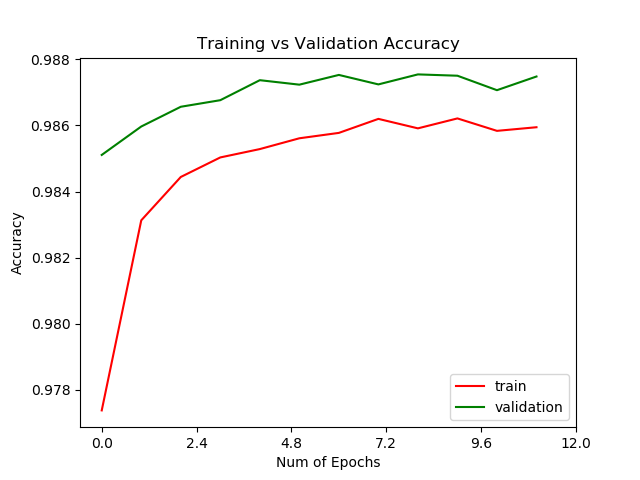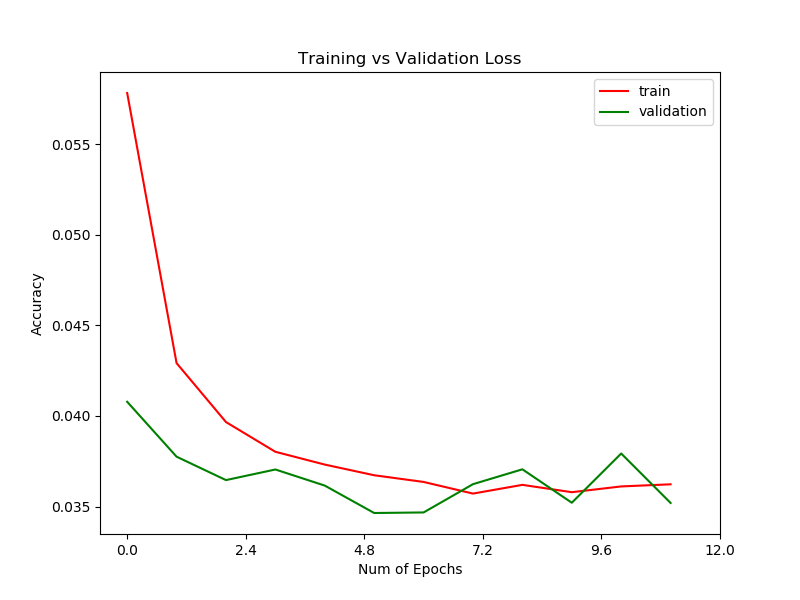As we can see in the charts above, the neural network learns quickly, getting good results.

#### Apply Precision-Recall

Precision (also called positive predictive value) is the fraction of relevant instances among the retrieved instances.

Recall (also known as sensitivity) is the fraction of relevant instances that have been retrieved over the total amount of relevant instances.

Both precision and recall are therefore based on an understanding and measure of relevance.

```# Apply Precision-Recall

from sklearn.metrics import recall_score
from sklearn.metrics import precision_score
from sklearn.metrics import f1_score

y_pred = model.predict(X_test)

# Convert Y_Test into 1D array
yy_true = [np.argmax(i) for i in Y_test]
print(yy_true)

yy_scores = [np.argmax(i) for i in y_pred]
print(yy_scores)

print("Recall: " + str(recall_score(yy_true, yy_scores, average='weighted')))
print("Precision: " + str(precision_score(yy_true, yy_scores, average='weighted')))
print("F1 Score: " + str(f1_score(yy_true, yy_scores, average='weighted')))```

#### Apply and Visualizing of the confusion matrix

Confusion Matrix also is known as an error matrix, is a specific table layout that allows visualization of the performance of an algorithm, typically a supervised learning one (in unsupervised learning it is usually called a matching matrix). Each row of the matrix represents the instances in a predicted class while each column represents the instances in an actual class (or vice versa).

```# Apply Confusion matrix

from sklearn.metrics import classification_report, confusion_matrix
Y_pred = model.predict(X_test, verbose=2)
y_pred = np.argmax(Y_pred, axis=1)

for ix in range(3):
print(ix, confusion_matrix(np.argmax(Y_test, axis=1), y_pred)[ix].sum())
cm = confusion_matrix(np.argmax(Y_test, axis=1), y_pred)
print(cm)

# Visualizing of confusion matrix
import seaborn as sn

df_cm = pd.DataFrame(cm, range(3), range(3))
plt.figure(figsize=(10,7))
sn.set(font_scale=1.4)
sn.heatmap(df_cm, annot=False)
sn.set_context("poster")
plt.xlabel("Predicted Label")
plt.ylabel("True Label")
plt.title("Confusion Matrix")
plt.savefig('Plots/confusionMatrix.png')
plt.show()```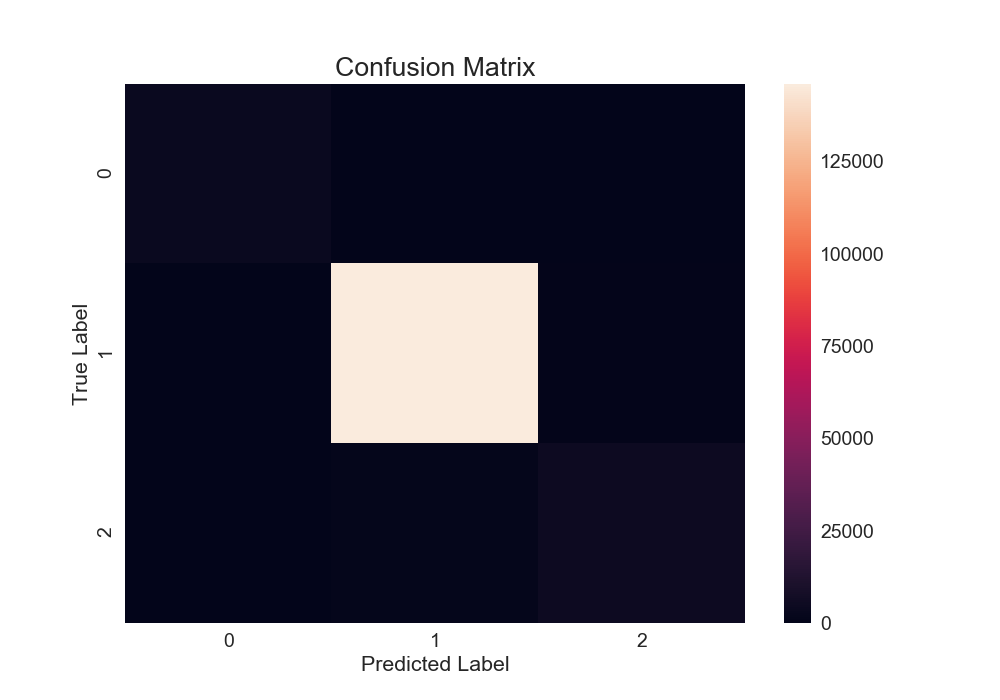#### Wordcloud

Word clouds (also known as text clouds or tag clouds) work in a simple way: the more a specific word appears in a source of textual data (such as a speech, blog post, or database), the bigger and bolder it appears in the word cloud.

```from wordcloud import WordCloud
from many_stop_words import get_stop_words

stop_words = get_stop_words('pl')

wordcloud = WordCloud(
background_color='white',
stopwords=stop_words,
max_words=200,
max_font_size=40,
random_state=42
).generate(str(dataset['description']))

print(wordcloud)
fig = plt.figure(1)
plt.imshow(wordcloud)
plt.axis('off')
plt.show()```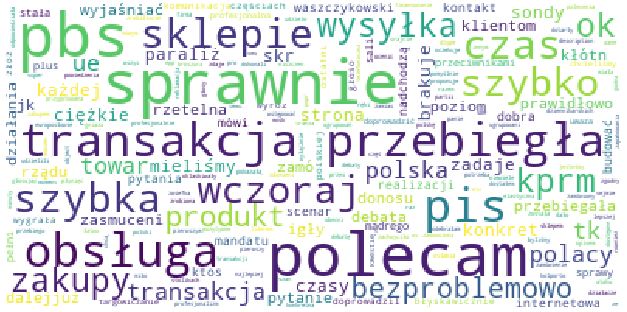The word cloud above shows the most common words in our dataset.

```# ROC Curve

from sklearn.metrics import roc_curve, auc
from scipy import interp
from itertools import cycle

# Compute ROC curve and ROC area for each class

n_classes = 3
lw = 2
fpr = dict()
tpr = dict()
roc_auc = dict()

for i in range(n_classes):
fpr[i], tpr[i], _ = roc_curve(np.array(pd.get_dummies(yy_true))[:, i], np.array(pd.get_dummies(yy_scores))[:, i])
roc_auc[i] = auc(fpr[i], tpr[i])

# Compute micro-average ROC curve and ROC area
fpr["micro"], tpr["micro"], _ = roc_curve(np.array(pd.get_dummies(yy_true))[:, i], np.array(pd.get_dummies(yy_scores))[:, i])
roc_auc["micro"] = auc(fpr["micro"], tpr["micro"])

# Compute macro-average ROC curve and ROC area

# First aggregate all false positive rates
all_fpr = np.unique(np.concatenate([fpr[i] for i in range(n_classes)]))

# Then interpolate all ROC curves at this points
mean_tpr = np.zeros_like(all_fpr)
for i in range(n_classes):
mean_tpr += interp(all_fpr, fpr[i], tpr[i])

# Finally average it and compute AUC
mean_tpr /= n_classes

fpr["macro"] = all_fpr
tpr["macro"] = mean_tpr
roc_auc["macro"] = auc(fpr["macro"], tpr["macro"])

# Plot all ROC curves
plt.figure(figsize=(8,5))
plt.plot(fpr["micro"], tpr["micro"],
label='micro-average ROC curve (area = {0:0.2f})'
''.format(roc_auc["micro"]),
color='deeppink', linestyle=':', linewidth=4)
plt.plot(fpr["macro"], tpr["macro"],
label='macro-average ROC curve (area = {0:0.2f})'
''.format(roc_auc["macro"]),
color='green', linestyle=':', linewidth=4)

colors = cycle(['aqua', 'darkorange', 'cornflowerblue'])
for i, color in zip(range(n_classes), colors):
plt.plot(fpr[i], tpr[i], color=color, lw=lw,
label='ROC curve of class {0} (area = {1:0.2f})'
''.format(i, roc_auc[i]))

plt.plot([0, 1], [0, 1], 'k--',color='red', lw=lw)
plt.xlim([0.0, 1.0])
plt.ylim([0.0, 1.05])
plt.xlabel('False Positive Rate')
plt.ylabel('True Positive Rate')
plt.legend(loc="lower right")
plt.savefig('Plots/ROCcurve.png')
plt.show()```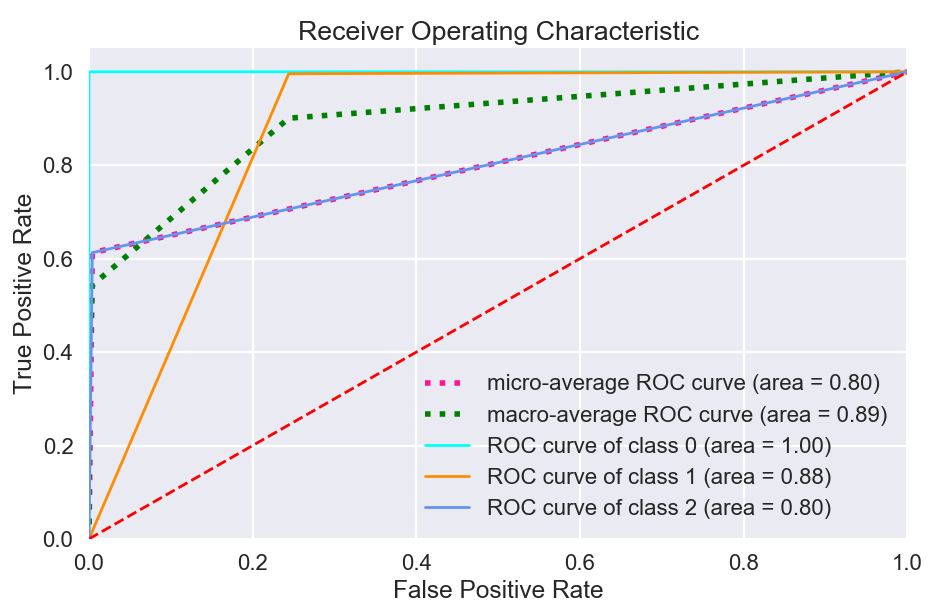The ROC curve is created by plotting the true positive rate (TPR) against the false positive rate (FPR) at various threshold settings. The true-positive rate is also known as sensitivityrecall.

### Results

Test Accuracy: 97,65%

Test Loss: 6,56%

Recall score: 0.9761942865880075

Precision score: 0.9757310701772396

F1 score: 0.9758853714533414

### Some statistics

• Our model was trained on a data set consisting of about 1,000,000 rows containing a minimum of 30 characters.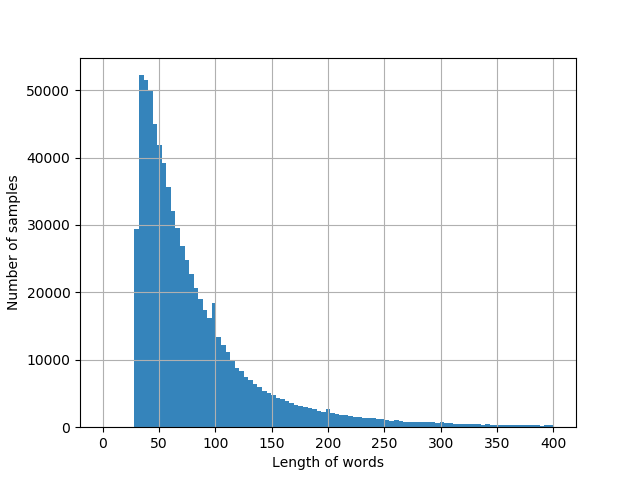• Training time:

For epochs = 12; 220 minutes on MacBook Pro i7, 2.5GHz, 16 GB RAM, 512 GB SSD.

### Summary

After working through this post you learned:

• What is a Sentiment Analysis
• How to use Keras library in NLP projects
• How to use Word2Vec
• How to preprocessing data for NLP projects
• How difficult is the Polish language in NLP
• How to implement LSTM
• What is a Word Embedding
• How to visualize on Wordcloud
• How to interpret confusion matrix, precision, recall, F1 score and ROC curve

### How to run Our project?

Go to README in project repository here.

### Resources

1. Mikolov, Tomas; et al. “Efficient Estimation of Word Representations in Vector Space”. arXiv:1301.3781
2. Twitter web scrapper & sentiment analysis of Polish government parties
3. Pre-trained word vectors of 30+ languages
4. An implementation of different neural networks to classify tweet’s sentiments
5. Predict Sentiment From Move Reviews Using Deep Learning
6. http://dsmodels.nlp.ipipan.waw.pl/
7. Chinese Shopping Reviews sentiment analysis
8. Prediction of Amazon review scores with a deep recurrent neural network using LSTM modules
9. Classify the sentiment of sentences from the Rotten Tomatoes dataset
10. Understanding LSTM Networks
11. https://keras.io/
Recommended Posts